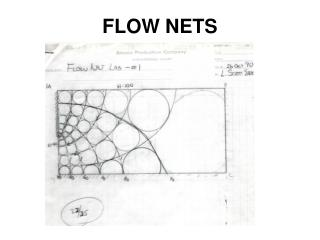DownloadDownload PresentationFLOW NETS

# FLOW NETS

Download Presentation## FLOW NETS

- - - - - - - - - - - - - - - - - - - - - - - - - - - E N D - - - - - - - - - - - - - - - - - - - - - - - - - - -
##### Presentation Transcript

1. FLOW NETS

2. Techniques for Finding “Solutions” to Groundwater Flow” • Inspection (intuition) • Graphical Techniques

3. Techniques for Finding “Solutions” to Groundwater Flow” • Inspection (intuition) • Graphical Techniques • Analog Models

4. Techniques for Finding “Solutions” to Groundwater Flow” • Inspection (intuition) • Graphical Techniques • Analog Models • Analytical Mathematical Techniques (Calculus)

5. Techniques for Finding “Solutions” to Groundwater Flow” • Inspection (intuition) • Graphical Techniques • Analog Models • Analytical Mathematical Techniques (Calculus) • Numerical Mathematical Techniques (Computers)

6. I. Introduction A. Overview

7. I. Introduction A. Overview • one of the most powerful tools for the analysis of groundwater flow.

8. I. Introduction A. Overview • one of the most powerful tools for the analysis of groundwater flow. • provides a solution to LaPlaces Equation for 2-D, steady state, boundary value problem.

9. I. Introduction A. Overview • one of the most powerful tools for the analysis of groundwater flow. • provides a solution to LaPlaces Equation for 2-D, steady state, boundary value problem. • To solve, need to know:

10. I. Introduction A. Overview • one of the most powerful tools for the analysis of groundwater flow. • provides a solution to LaPlaces Equation for 2-D, steady state, boundary value problem. • To solve, need to know: • have knowledge of the region of flow

11. I. Introduction A. Overview • one of the most powerful tools for the analysis of groundwater flow. • provides a solution to LaPlaces Equation for 2-D, steady state, boundary value problem. • To solve, need to know: • have knowledge of the region of flow • boundary conditions along the perimeter of the region

12. To solve, need to know: • have knowledge of the region of flow • boundary conditions along the perimeter of the region • spatial distribution of hydraulic head in region.

13. Composed of 2 sets of lines • equipotential lines (connect points of equal hydraulic head) • flow lines (pathways of water as it moves through the aquifer.

14. Composed of 2 sets of lines • equipotential lines (connect points of equal hydraulic head) • flow lines (pathways of water as it moves through the aquifer. d2h + d2h = 0 gives the rate of change of dx2 dy2 h in 2 dimensions

15. II. Assumptions Needed For Flow Net Construction

16. Assumptions Needed For Flow Net Construction • Aquifer is homogeneous, isotropic • Aquifer is saturated

17. Assumptions Needed For Flow Net Construction • Aquifer is homogeneous, isotropic • Aquifer is saturated • There is no change in head with time

18. Assumptions Needed For Flow Net Construction • Aquifer is homogeneous, isotropic • Aquifer is saturated • There is no change in head with time • Soil and water are incompressible

19. Assumptions Needed For Flow Net Construction • Aquifer is homogeneous, isotropic • Aquifer is saturated • there is no change in head with time • soil and water are incompressible • Flow is laminar, and Darcys Law is valid

20. Assumptions Needed For Flow Net Construction • Aquifer is homogeneous, isotropic • Aquifer is saturated • there is no change in head with time • soil and water are incompressible • flow is laminar, and Darcys Law is valid • All boundary conditions are known.

21. III. Boundaries

22. III. Boundaries A. Types

23. III. Boundaries • Types 1. Impermeable 2. Constant Head 3. Water Table

24. III. Boundaries • Types 1. Impermeable 2. Constant Head 3. Water Table

25. B. Calculating Discharge Using Flow Nets III. Boundaries Q’ = Kph f Where: Q’ = Discharge per unit depth of flow net (L3/t/L) K = Hydraulic Conductivity (L/t) p = number of flow tubes h = head loss (L) f = number of equipotential drops

26. IV. Refraction of Flow Lines

27. IV. Refraction of Flow Lines • The derivation • The general relationships • An example problem

28. IV. Flow Nets: Isotropic, Heterogeneous Types • “Reminder” of the conditions needed to draw a flow net for homogeneous, isotropic conditions • An Example of Iso, Hetero